/* styles */ Yes, we are participating in Quilter's Trek this year (formerly Row by Row Experience) Yes, the hounds are in the row this year Yes, Laura is way behind this year BUT... this year we can mail kits starting now! Well, for Cotton Patch it will be mailing early next week, but the point is we are allowed to mail kits from the beginning! Free patterns are still an instore pickup, but we are not open for walk ins, so that will be put on hold for a little bit yet. So, do you want to see our row this year???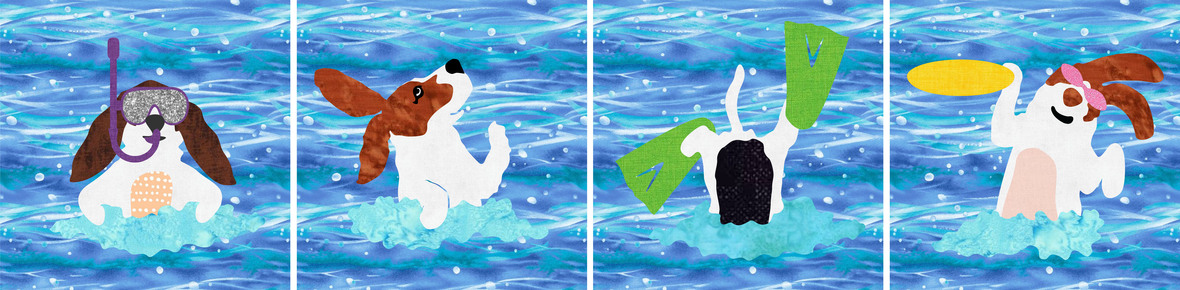/* styles */ It's Dippin' Dawgs! The theme this year is the color blue Shops were to design 4 blocks that finish at 9" square Blocks can be put together as a row, a square, or used individually. So Freddie, Maggie, Stanley and Lulu are having a day at the beach!
 table div table+table+table+table+table+table div table{width:100%;padding:0}table div table+table+table+table+table+table div table img{width:96.23%;padding:0;float:none}table div table+table+table+table+table+table div table td{width:100%;padding:0 1.88% 18px}/* styles */# Feeling Wicked

If you like skulls and spiders and creepy things on your Halloween fabric, then Wicked is the line for you!
Great panel with many possibilities and coordinates to go with it.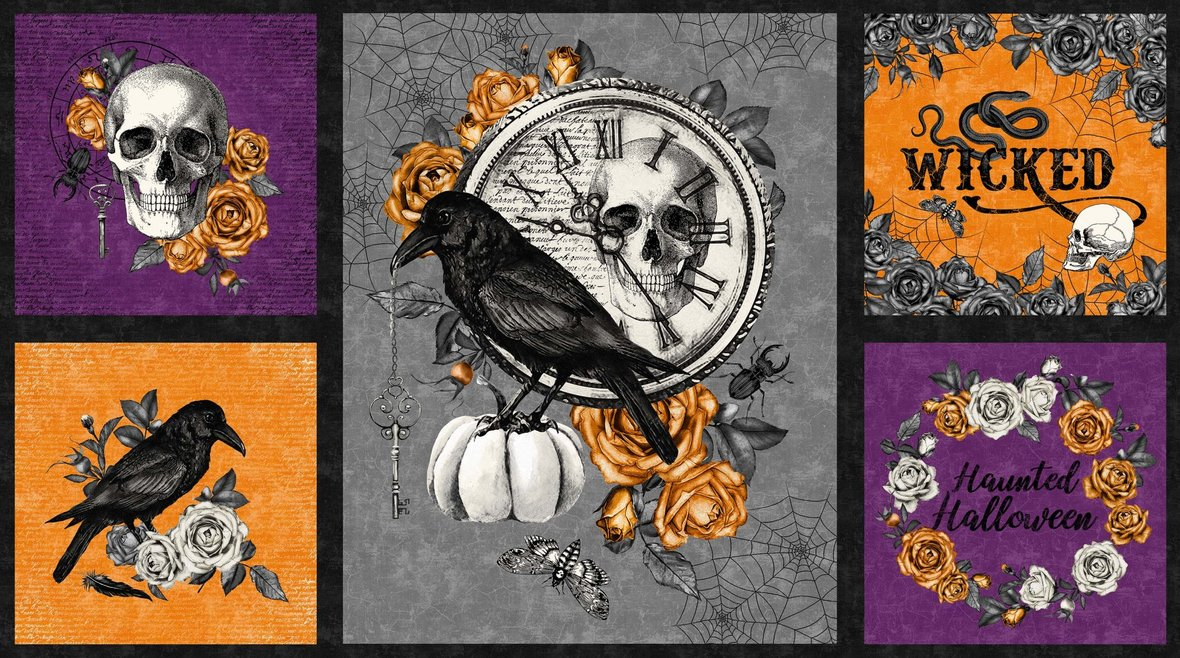table div table+table+table+table+table+table+table+table+table+table div table{width:100%;padding:0}table div table+table+table+table+table+table+table+table+table+table div table img{width:96.23%;padding:0;float:none}table div table+table+table+table+table+table+table+table+table+table div table td{width:100%;padding:0 1.88% 18px}/* styles */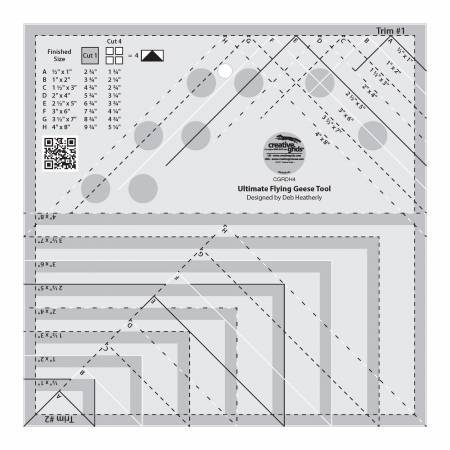# New Goodies!

We are always looking for new patterns, books and tools for you!
Quilt as you go placemats
Cute Kimberbell Buttons
Flying Geese Ruler that makes 8 sizes!

 table div table+table+table+table+table+table+table+table+table+table+table+table+table div table{width:100%;padding:0}table div table+table+table+table+table+table+table+table+table+table+table+table+table div table img{width:96.23%;padding:0;float:none}table div table+table+table+table+table+table+table+table+table+table+table+table+table div table td{width:100%;padding:0 1.88% 18px}/* styles *//* styles */ Psssst ... have you heard? Kimberbell's Love Notes Mystery Quilt is happening at Cotton Patch this summer! Available for both sewing and machine embroidery, the Love Notes Mystery Quilt is an exciting way to experience the joy of creativity™ with other stitching enthusiasts starting August 3rd. Kits include Kimberbell fabrics and Kimberbell embellishments to add that special touch in a super cute custom Kimberbell box, plus your clues are sent to you for 5 weeks in love notes! Kits are limited, so reserve yours today, and each participant will be sent a free project in June to stitch while we wait for the mystery to start.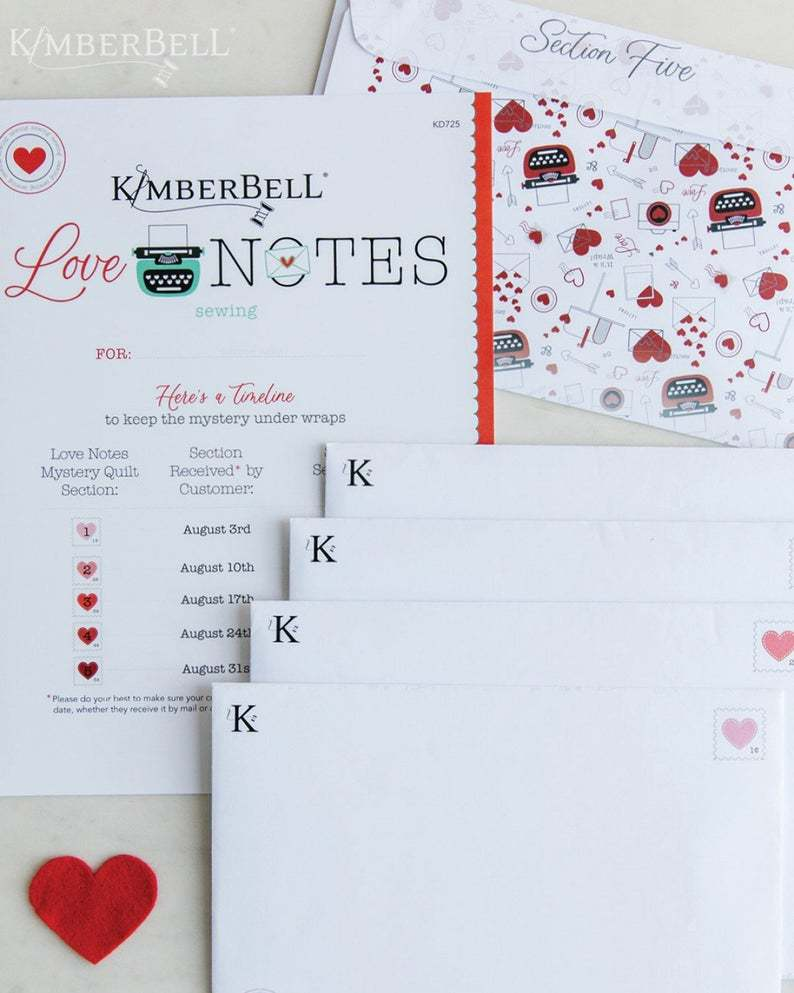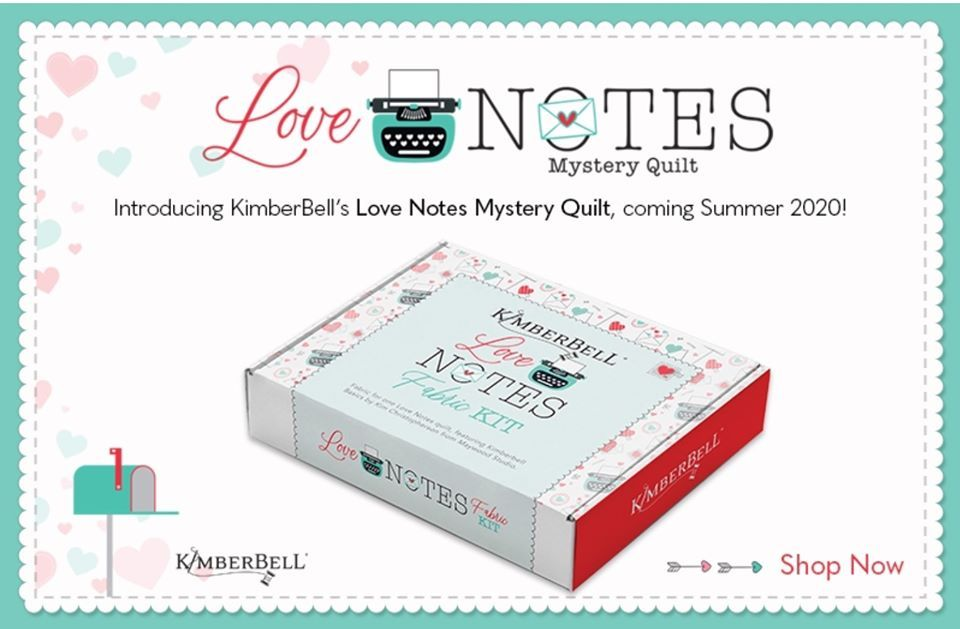table div table+table+table+table+table+table+table+table+table+table+table+table+table+table+table+table+table+table div table{width:100%;padding:0}table div table+table+table+table+table+table+table+table+table+table+table+table+table+table+table+table+table+table div table img{width:96.23%;padding:0;float:none}table div table+table+table+table+table+table+table+table+table+table+table+table+table+table+table+table+table+table div table td{width:100%;padding:0 1.88% 18px}/* styles */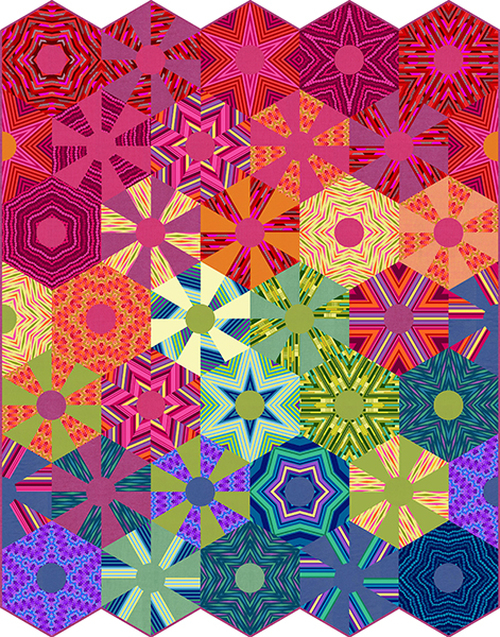# All Lined Up!

This beauty of a quilt is made up of hexagons from an assortment of striped fabrics - and get this - it is a digitally printed quilt top you can purchase!
That's right - no piecing!
This quilt top will deliver in September and we are taking preorders now.
Quantities are limited, so we likely won't be able to order extra, so if you want it, place a preorder.

 table div table+table+table+table+table+table+table+table+table+table+table+table+table+table+table+table+table+table+table+table+table div table{width:100%;padding:0}table div table+table+table+table+table+table+table+table+table+table+table+table+table+table+table+table+table+table+table+table+table div table img{width:96.23%;padding:0;float:none}table div table+table+table+table+table+table+table+table+table+table+table+table+table+table+table+table+table+table+table+table+table div table td{width:100%;padding:0 1.88% 18px}/* styles */## Mask Templates are in Stock!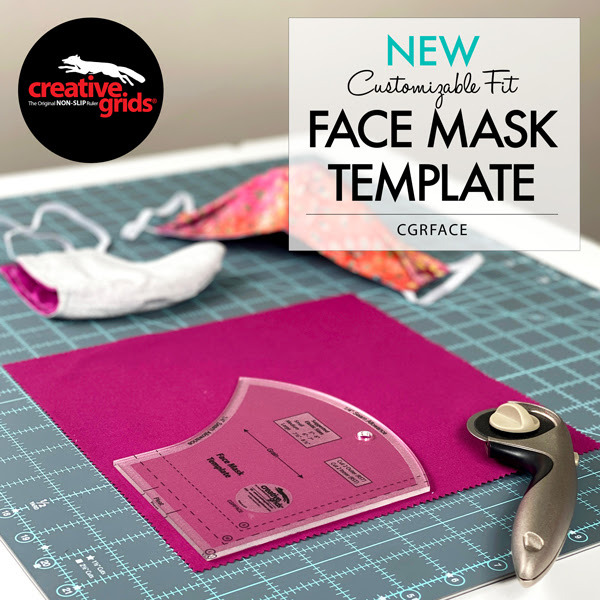New from Creative Grids, a 3-in-1 template for cutting out masks in 3 different sizes!

 table div table+table+table+table+table+table+table+table+table+table+table+table+table+table+table+table+table+table+table+table+table+table+table+table+table div table{width:100%;padding:0}table div table+table+table+table+table+table+table+table+table+table+table+table+table+table+table+table+table+table+table+table+table+table+table+table+table div table img{width:96.23%;padding:0;float:none}table div table+table+table+table+table+table+table+table+table+table+table+table+table+table+table+table+table+table+table+table+table+table+table+table+table div table td{width:100%;padding:0 1.88% 18px}/* styles */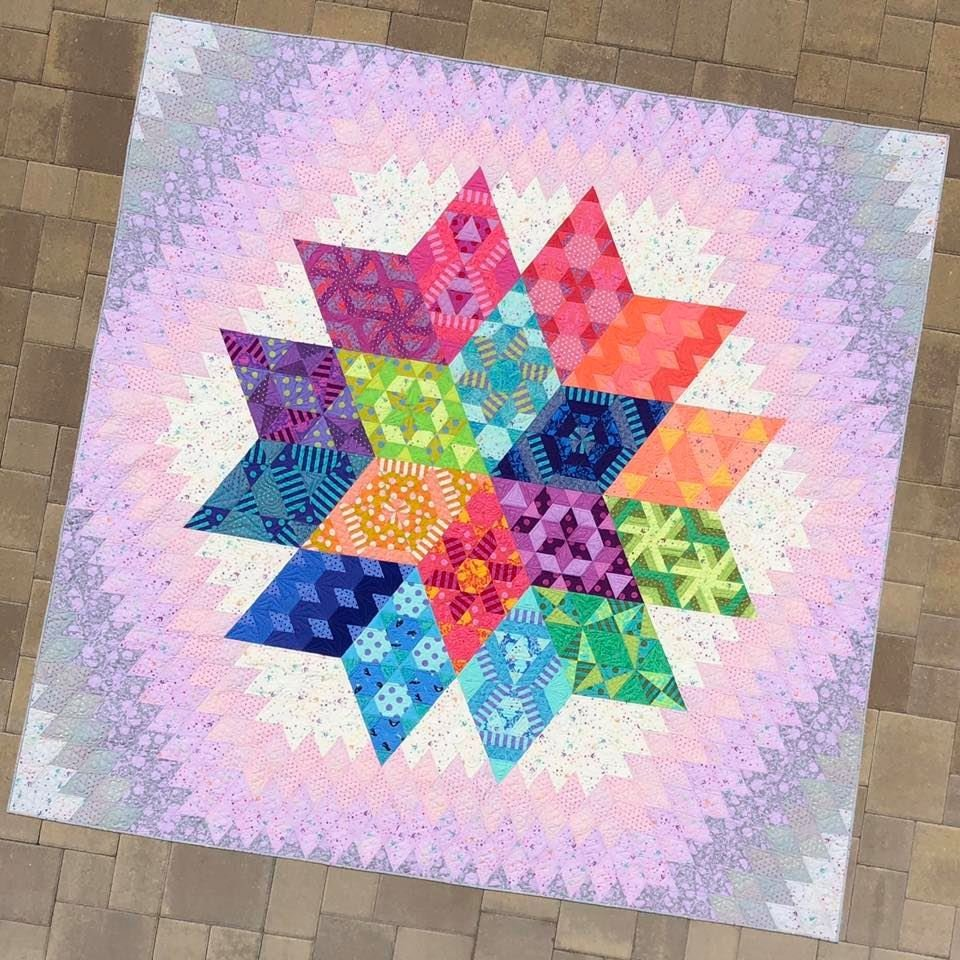# Nebula Block of the Month

Reserve your spot in this 10 month program beginning in January 2021!

Create 9 unique blocks using, are you ready for this, 74 different Tula Pink fabrics and 7 Tula Pink solids!

Pattern is by Jaybird Quilts and there are NO Y-Seams!

Sign up before July 31 and receive an exclusive Tula Pink project bag with a Nebula Label!

 table div table+table+table+table+table+table+table+table+table+table+table+table+table+table+table+table+table+table+table+table+table+table+table+table+table+table+table+table div table{width:100%;padding:0}table div table+table+table+table+table+table+table+table+table+table+table+table+table+table+table+table+table+table+table+table+table+table+table+table+table+table+table+table div table img{width:96.23%;padding:0;float:none}table div table+table+table+table+table+table+table+table+table+table+table+table+table+table+table+table+table+table+table+table+table+table+table+table+table+table+table+table div table td{width:100%;padding:0 1.88% 18px}/* styles */## Finish Up Your Sewing Sewcial Quilt!

 /* styles */ The block sales are over, now it's time to finish up those Sewing Sewcial quilts! Have you picked your finishing kit or are you doing your own thing? We have a limited amount of yardage available of these exclusive Sewing Sewcial Batiks - once they are gone, that's it!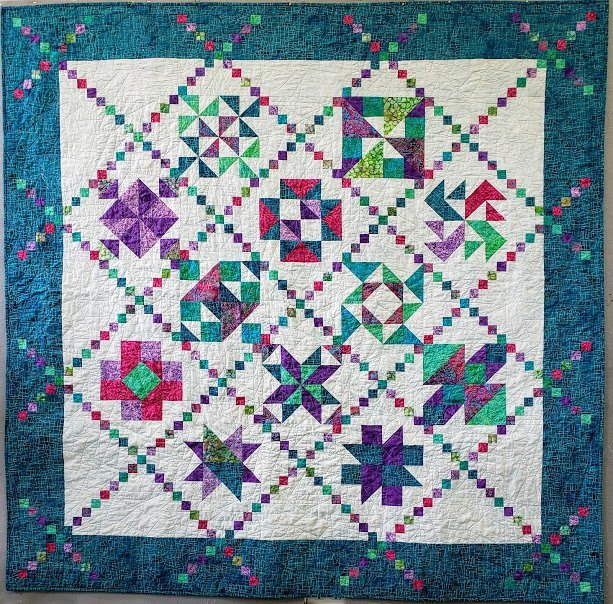# This is our finishing kit!

The fabrics needed for the finishing kit will be delivered this week - kits will be available at the end of this week!

 table div table+table+table+table+table+table+table+table+table+table+table+table+table+table+table+table+table+table+table+table+table+table+table+table+table+table+table+table+table+table+table+table+table div table{width:100%;padding:0}table div table+table+table+table+table+table+table+table+table+table+table+table+table+table+table+table+table+table+table+table+table+table+table+table+table+table+table+table+table+table+table+table+table div table img{width:96.23%;padding:0;float:none}table div table+table+table+table+table+table+table+table+table+table+table+table+table+table+table+table+table+table+table+table+table+table+table+table+table+table+table+table+table+table+table+table+table div table td{width:100%;padding:0 1.88% 18px}/* styles */# FB Live is the place to be Sunday at 7pm!

You will be the 1st to see new fabrics, notions, kits and other great things that you need to know about!

## We put together fun kits that often sell out that very night - you don't want to miss out!

There will be prizes, live demos and special pricing!

You will be able to purchase during the Live Broadcast and we can ship - sort of like Stuff Your Stocking but all in one night.
If you miss the FB Live - not to worry!

## You can view the replay on our FB Page AND you can still purchase at the special prices until 9pm Monday night!

We will go "live" at 6:55 and see what the hounds are up to, then
start the Soiree at 7pm (Eastern)

## Join me for a fun evening!

 table div table+table+table+table+table+table+table+table+table+table+table+table+table+table+table+table+table+table+table+table+table+table+table+table+table+table+table+table+table+table+table+table+table+table+table+table+table+table div table{width:100%;padding:0}table div table+table+table+table+table+table+table+table+table+table+table+table+table+table+table+table+table+table+table+table+table+table+table+table+table+table+table+table+table+table+table+table+table+table+table+table+table+table div table img{width:96.23%;padding:0;float:none}table div table+table+table+table+table+table+table+table+table+table+table+table+table+table+table+table+table+table+table+table+table+table+table+table+table+table+table+table+table+table+table+table+table+table+table+table+table+table div table td{width:100%;padding:0 1.88% 18px}/* styles */# Meet me in the Club House!

Cotton Patch Club House is a new FaceBook group and we want you to be a part of it!
You will find:
An online community for friends of Cotton Patch Quilt Shop!
A place to chat and share photos of your projects.
A place to ask quilty questions, share tips and demos
A place for you to find sneak peeks and early access to items on our FaceBook Live Sunday Soiree!

 table div table+table+table+table+table+table+table+table+table+table+table+table+table+table+table+table+table+table+table+table+table+table+table+table+table+table+table+table+table+table+table+table+table+table+table+table+table+table+table+table+table div table{width:100%;padding:0}table div table+table+table+table+table+table+table+table+table+table+table+table+table+table+table+table+table+table+table+table+table+table+table+table+table+table+table+table+table+table+table+table+table+table+table+table+table+table+table+table+table div table img{width:96.23%;padding:0;float:none}table div table+table+table+table+table+table+table+table+table+table+table+table+table+table+table+table+table+table+table+table+table+table+table+table+table+table+table+table+table+table+table+table+table+table+table+table+table+table+table+table+table div table td{width:100%;padding:0 1.88% 18px}/* styles *//* styles */ Although the Stay at Home Order has been lifted in Florida, the storefront at Cotton Patch will remained closed to shopping for now. An opening date is still to be determined. Our website is open 24/7 and we are filling orders for curbside pickup or shipping right to your door! To best serve you, all orders should be placed through the website and not over the phone. Payment on the website is more secure than reading your credit card numbers over the phone and less chance for errors on items/quantity. Curbside pickup is available 10am - 2pm Tuesday through Saturday. You will get an email telling you when you order is ready for pickup. Call us from the parking lot and we will bring your purchases out to you. Thank you for your continued support with online orders and curbside pickup - we appreciate your business! We miss your smiling faces and look forward to welcoming you back soon! Laura
 table div table+table+table+table+table+table+table+table+table+table+table+table+table+table+table+table+table+table+table+table+table+table+table+table+table+table+table+table+table+table+table+table+table+table+table+table+table+table+table+table+table+table+table div table{width:100%;padding:0}table div table+table+table+table+table+table+table+table+table+table+table+table+table+table+table+table+table+table+table+table+table+table+table+table+table+table+table+table+table+table+table+table+table+table+table+table+table+table+table+table+table+table+table div table img{width:96.23%;padding:0;float:none}table div table+table+table+table+table+table+table+table+table+table+table+table+table+table+table+table+table+table+table+table+table+table+table+table+table+table+table+table+table+table+table+table+table+table+table+table+table+table+table+table+table+table+table div table td{width:100%;padding:0 1.88% 18px}/* styles *//* styles */ What's up Stanley?
 table.module-44{width:56.79%;padding:0}table div table+table+table+table+table+table+table+table+table+table+table+table+table+table+table+table+table+table+table+table+table+table+table+table+table+table+table+table+table+table+table+table+table+table+table+table+table+table+table+table+table+table+table+table+table div table{width:56.79%;float:none;margin-left:auto;margin-right:auto;padding:0}table div table+table+table+table+table+table+table+table+table+table+table+table+table+table+table+table+table+table+table+table+table+table+table+table+table+table+table+table+table+table+table+table+table+table+table+table+table+table+table+table+table+table+table+table+table div table a{border:0 none;text-decoration:none}table div table+table+table+table+table+table+table+table+table+table+table+table+table+table+table+table+table+table+table+table+table+table+table+table+table+table+table+table+table+table+table+table+table+table+table+table+table+table+table+table+table+table+table+table+table div table img{width:100%!important;border:0 none;text-decoration:none}table div table+table+table+table+table+table+table+table+table+table+table+table+table+table+table+table+table+table+table+table+table+table+table+table+table+table+table+table+table+table+table+table+table+table+table+table+table+table+table+table+table+table+table+table+table div table td{width:100%;padding:0}/* styles */
 Like   Pin   +1